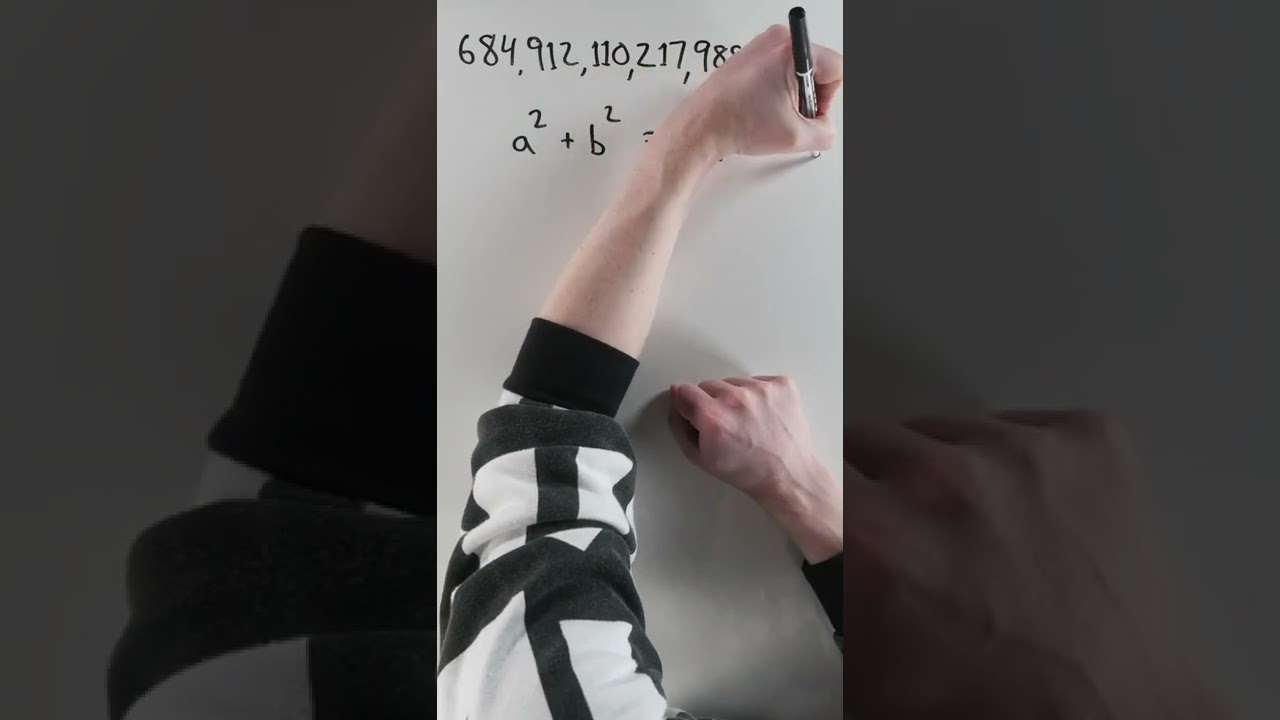Vsauce

# Pi Hidden in a Huge Triangle #shorts

You all know the Pythagorean theorem, but what does a right triangle with a hypotenuse of 684,912,110,217,988,900 reveal about Pi? A lot, once you figure out the other two sides.

If the square root of 684,912,110,217,988,900 yields 827,594,170, and that equals a^2 + b^2, we can find the ratio of a to b. And the result is an extremely close approximation to pi that matches to 9 digits. Thanks, 684,912,110,217,988,900!

#shorts